Data Series 119

Seismic Profiles

Home | Acronyms | Contents | Navigation | Profiles | Logs | Metadata | Software

This page contains thumbnail images and links to full-size printable and interactive seismic reflection profiles. The printable profiles are GIF images that were filtered and gained using Seismic Unix software. These images are stored in the PRINTABLE folder. Refer to the Software page for details about the processing and examples of the processing scripts.

The processed SEG-Y data were then exported to CTI SonarWeb software to produce an interactive version of the profile that allows the user to obtain a geographic location and depth from the profile for a cursor position. This information is displayed in the status bar of the browser. The interactive profiles, which have not been corrected for the offset between the shotpoint and GPS antenna (see (fig. 1), are stored in the INTERACTIVE folder.

Warning! The only supported web browsers that properly display all features of the interactive profiles are Internet Explorer 6 for Windows and Firefox 1.5 for all platforms. Caution–other browsers may result in spurious or no information given in the status window. Firefox may be downloaded at http://www.mozilla.org/products/firefox.

Note: The Web version of this archive does not contain the SEG-Y Trace files. These files are very large and would require extremely long download times. To obtain the complete DVD archive, contact USGS Information Services at 1-888-ASK-USGS.

Browser setup for viewing interactive profiles:
For all browsers, the status bar and JavaScript must be enabled. Firefox also requires "Change status bar text" to be checked under Advanced JavaScript Options.

Table 2 lists trackline statistics.

SEG-Y Format

*NOTE: Navigation is missing for the start of lines 04b16a and 04b22.

Line: 04b01 1073 traces:
Start of line: 04b01 SHOT=1 day=61 hour=17 minute=2 second=36
End of line: 04b01 SHOT=1073 day=61 hour=17 minute=7 second=4
INTERACTIVE | PRINTABLE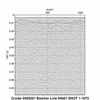Line: 04b02 18995 traces:
Start of line: 04b02 SHOT=1 day=61 hour=17 minute=18 second=19
End of line: 04b02 SHOT=18995 day=61 hour=18 minute=37 second=28
INTERACTIVE | PRINTABLE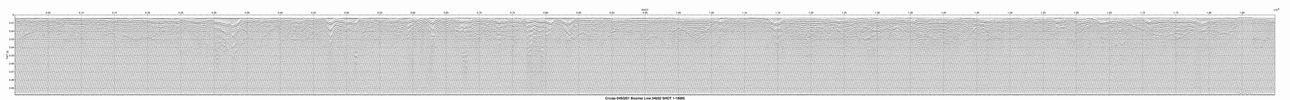Line: 04b03 9920 traces:
Start of line: 04b03 SHOT=1 day=61 hour=18 minute=37 second=29
End of line: 04b03 SHOT=9920 day=61 hour=19 minute=18 second=49
INTERACTIVE | PRINTABLE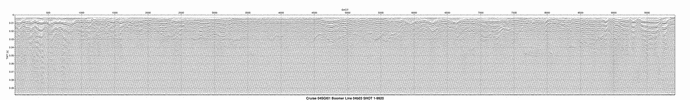Line: 04b04a 20000 traces:
Start of line: 04b04a SHOT=1 day=61 hour=19 minute=43 second=36
End of line: 04b04a SHOT=20000 day=61 hour=21 minute=6 second=57
INTERACTIVE | PRINTABLE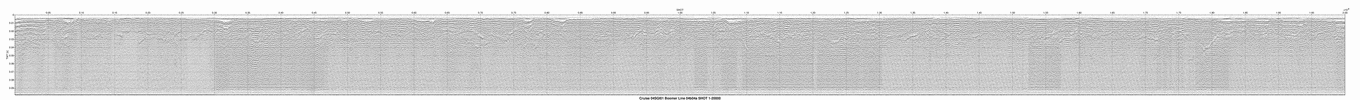Line: 04b04b 11616 traces:
Start of line: 04b04b SHOT=1 day=61 hour=21 minute=6 second=57
End of line: 04b04b SHOT=11616 day=61 hour=21 minute=55 second=22
INTERACTIVE | PRINTABLE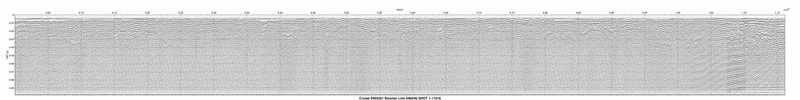Line: 04b05 3274 traces:
Start of line: 04b05 SHOT=1 day=61 hour=21 minute=55 second=22
End of line: 04b05 SHOT=3274 day=61 hour=22 minute=9 second=0
INTERACTIVE | PRINTABLE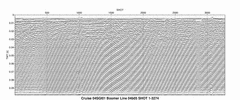Line: 04b05a 317 traces:
Start of line: 04b05a SHOT=1 day=61 hour=22 minute=14 second=33
End of line: 04b05a SHOT=317 day=61 hour=22 minute=15 second=52
INTERACTIVE | PRINTABLE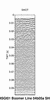Line: test 455 traces:
Start of line: test SHOT=1 day=62 hour=14 minute=21 second=57
End of line: test SHOT=455 day=62 hour=14 minute=23 second=50
INTERACTIVE | PRINTABLE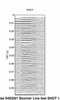Line: 04b06 9348 traces:
Start of line: 04b06 SHOT=1 day=62 hour=15 minute=32 second=2
End of line: 04b06 SHOT=9348 day=62 hour=16 minute=10 second=59
INTERACTIVE | PRINTABLE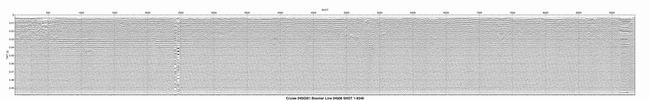Line: 04b07 14681 traces:
Start of line: 04b07 SHOT=1 day=62 hour=16 minute=16 second=16
End of line: 04b07 SHOT=14681 day=62 hour=17 minute=17 second=26
INTERACTIVE | PRINTABLE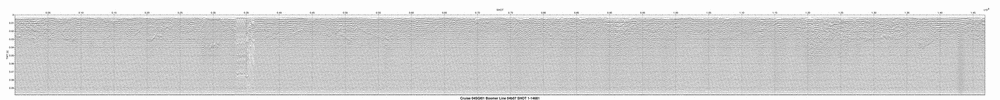Line: 04b08 5775 traces:
Start of line: 04b08 SHOT=1 day=62 hour=17 minute=17 second=26
End of line: 04b08 SHOT=5775 day=62 hour=17 minute=41 second=30
INTERACTIVE | PRINTABLE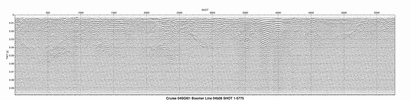Line: 04b09 11102 traces:
Start of line: 04b09 SHOT=1 day=62 hour=17 minute=47 second=57
End of line: 04b09 SHOT=11102 day=62 hour=18 minute=34 second=12
INTERACTIVE | PRINTABLE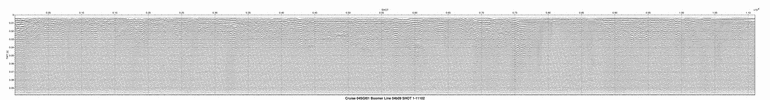Line: 04b10a 20000 traces:
Start of line: 04b10a SHOT=1 day=62 hour=18 minute=36 second=39
End of line: 04b10a SHOT=20000 day=62 hour=19 minute=59 second=58
INTERACTIVE | PRINTABLE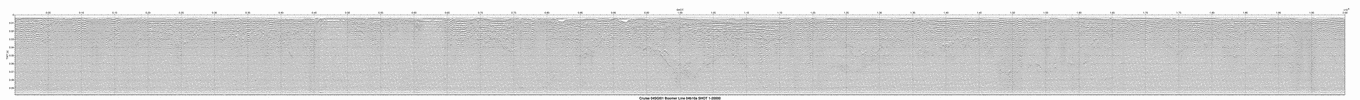Line: 04b10b 14386 traces:
Start of line: 04b10b SHOT=1 day=62 hour=19 minute=59 second=59
End of line: 04b10b SHOT=14386 day=62 hour=20 minute=59 second=56
INTERACTIVE | PRINTABLE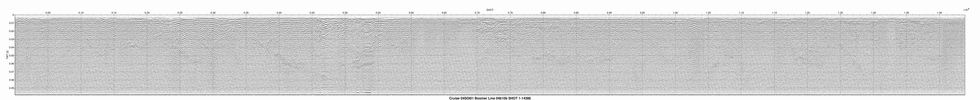Line: 04b11 16960 traces:
Start of line: 04b11 SHOT=1 day=62 hour=20 minute=59 second=56
End of line: 04b11 SHOT=16960 day=62 hour=22 minute=10 second=35
INTERACTIVE | PRINTABLE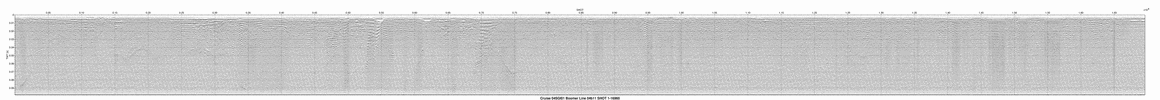Line: 04b12 7109 traces:
Start of line: 04b12 SHOT=1 day=63 hour=14 minute=56 second=42
End of line: 04b12 SHOT=7109 day=63 hour=15 minute=26 second=19
INTERACTIVE | PRINTABLE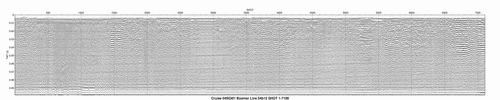Line: 04b13 1798 traces:
Start of line: 04b13 SHOT=1 day=63 hour=15 minute=51 second=36
End of line: 04b13 SHOT=1798 day=63 hour=15 minute=59 second=5
INTERACTIVE | PRINTABLE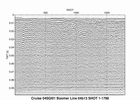Line: 04b14 1114 traces:
Start of line: 04b14 SHOT=1 day=63 hour=16 minute=13 second=19
End of line: 04b14 SHOT=1114 day=63 hour=16 minute=17 second=59
INTERACTIVE | PRINTABLE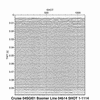Line: 04b14a 6897 traces:
Start of line: 04b14a SHOT=1 day=63 hour=16 minute=27 second=11
End of line: 04b14a SHOT=6897 day=63 hour=16 minute=55 second=55
INTERACTIVE | PRINTABLE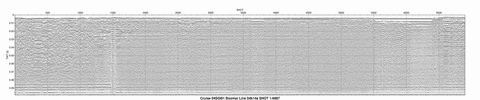Line: 04b15 828 traces:
Start of line: 04b15 SHOT=1 day=63 hour=16 minute=55 second=55
End of line: 04b15 SHOT=828 day=63 hour=16 minute=59 second=23
INTERACTIVE | PRINTABLE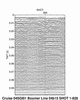Line: 04b16a 20000 traces:
Start of line: 04b16a SHOT=1 day=63 hour=* minute=* second=*
End of line: 04b16a SHOT=20000 day=63 hour=20 minute=11 second=22
INTERACTIVE | PRINTABLE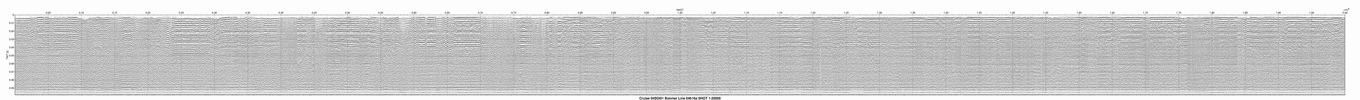Line: 04b16b 16450 traces:
Start of line: 04b16b SHOT=1 day=63 hour=20 minute=11 second=22
End of line: 04b16b SHOT=16450 day=63 hour=21 minute=22 second=52
INTERACTIVE | PRINTABLE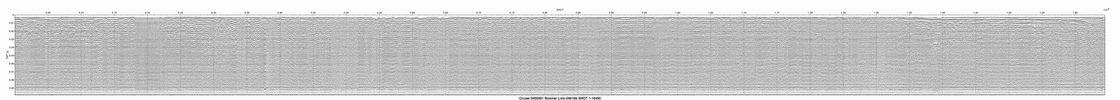Line: 04b17 8529 traces:
Start of line: 04b17 SHOT=1 day=63 hour=21 minute=22 second=52
End of line: 04b17 SHOT=8529 day=63 hour=21 minute=58 second=23
INTERACTIVE | PRINTABLE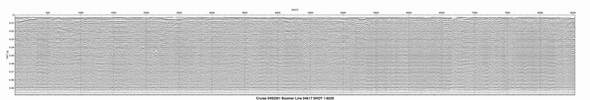Line: 04b18 10460 traces:
Start of line: 04b18 SHOT=1 day=63 hour=22 minute=3 second=31
End of line: 04b18 SHOT=10460 day=63 hour=22 minute=47 second=6
INTERACTIVE | PRINTABLE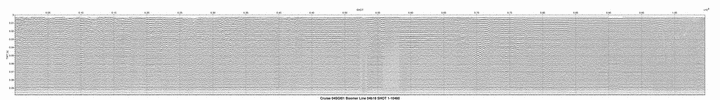Line: 04b19 3014 traces:
Start of line: 04b19 SHOT=1 day=63 hour=22 minute=52 second=39
End of line: 04b19 SHOT=3014 day=63 hour=23 minute=5 second=13
INTERACTIVE | PRINTABLE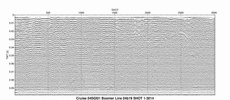Line: 04b20a 20000 traces:
Start of line: 04b20a SHOT=1 day=64 hour=14 minute=6 second=54
End of line: 04b20a SHOT=20000 day=64 hour=15 minute=30 second=15
INTERACTIVE | PRINTABLE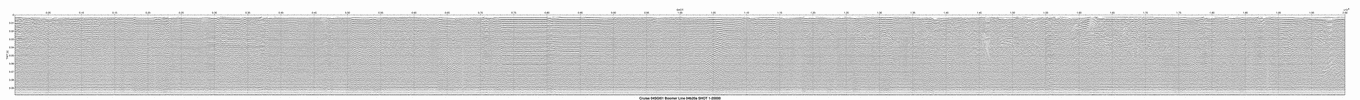Line: 04b20b 10733 traces:
Start of line: 04b20b SHOT=1 day=64 hour=15 minute=30 second=16
End of line: 04b20b SHOT=10733 day=64 hour=16 minute=14 second=59
INTERACTIVE | PRINTABLE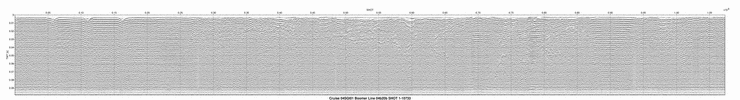Line: 04b21 17876 traces:
Start of line: 04b21 SHOT=1 day=64 hour=16 minute=14 second=59
End of line: 04b21 SHOT=17876 day=64 hour=17 minute=29 second=29
INTERACTIVE | PRINTABLE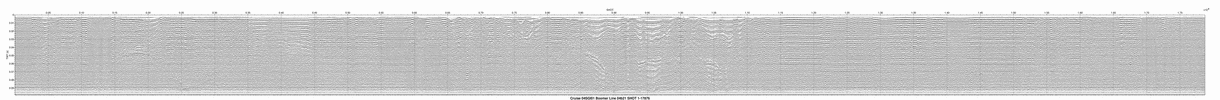Line: 04b22 10113 traces:
Start of line: 04b22 SHOT=1 day=64 hour=* minute=* second=*
End of line: 04b22 SHOT=10113 day=64 hour=18 minute=22 second=20
INTERACTIVE | PRINTABLE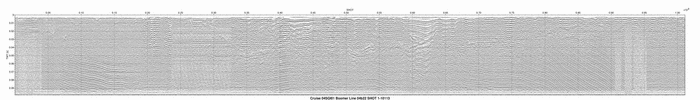Line: 04b23 7734 traces:
Start of line: 04b23 SHOT=1 day=64 hour=18 minute=27 second=11
End of line: 04b23 SHOT=7734 day=64 hour=18 minute=59 second=25
INTERACTIVE | PRINTABLE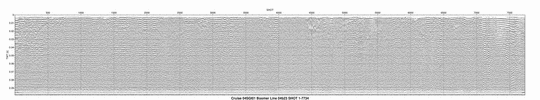Line: 04b24 7095 traces:
Start of line: 04b24 SHOT=1 day=64 hour=19 minute=17 second=32
End of line: 04b24 SHOT=7095 day=64 hour=19 minute=47 second=6
INTERACTIVE | PRINTABLE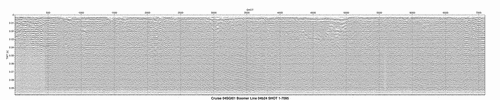Line: 04b25 5578 traces:
Start of line: 04b25 SHOT=1 day=64 hour=19 minute=52 second=43
End of line: 04b25 SHOT=5578 day=64 hour=20 minute=15 second=57
INTERACTIVE | PRINTABLE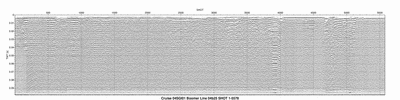Line: 04b26 6870 traces:
Start of line: 04b26 SHOT=1 day=64 hour=20 minute=29 second=50
End of line: 04b26 SHOT=6870 day=64 hour=20 minute=58 second=28
INTERACTIVE | PRINTABLE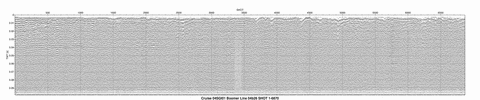Line: 04b27a 20000 traces:
Start of line: 04b27a SHOT=1 day=65 hour=14 minute=4 second=29
End of line: 04b27a SHOT=20000 day=65 hour=15 minute=27 second=50
INTERACTIVE | PRINTABLE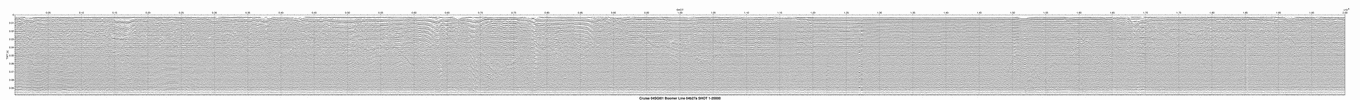Line: 04b27b 10773 traces:
Start of line: 04b27b SHOT=1 day=65 hour=15 minute=27 second=50
End of line: 04b27b SHOT=10773 day=65 hour=16 minute=12 second=44
INTERACTIVE | PRINTABLE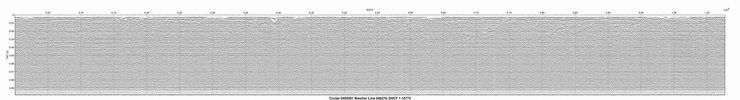Line: 04b28 4468 traces:
Start of line: 04b28 SHOT=1 day=65 hour=16 minute=12 second=45
End of line: 04b28 SHOT=4468 day=65 hour=16 minute=31 second=21
INTERACTIVE | PRINTABLE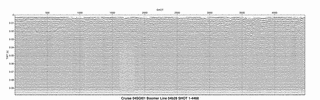Line: 04b30 2159 traces:
Start of line: 04b30 SHOT=1 day=65 hour=17 minute=50 second=33
End of line: 04b30 SHOT=2159 day=65 hour=17 minute=59 second=33
INTERACTIVE | PRINTABLE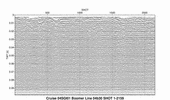Line: 04b31 11860 traces:
Start of line: 04b31 SHOT=1 day=65 hour=20 minute=7 second=29
End of line: 04b31 SHOT=11860 day=65 hour=20 minute=58 second=22
INTERACTIVE | PRINTABLE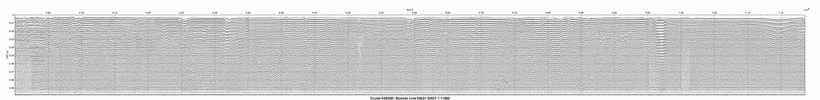Home | Acronyms | Contents | Navigation | Profiles | Logs | Metadata | Software